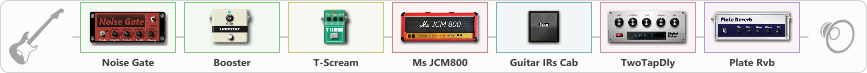# Solo variation

Discussion in 'ToneLib-GFX presets' started by tod, Feb 11, 2020.

1. Solo variation

Preset name: Solo variation

Bridge & both pick-ups

Effects chain:Effect: "Noise Gate" (Dynamics / Filter), active - "yes"
{
"Mode" = Manual
"Depth" = 74
"Threshold" = 43
"Attack" = 0
"Hold" = 5
"Decay" = 250
}

Effect: "Booster" (Dynamics / Filter), active - "yes"
{
"Gain" = 25
}

Effect: "T-Scream" (Overdrive / Distortion), active - "yes"
{
"Drive" = 100
"Tone" = 42
"Level" = 74
}

Effect: "Ms JCM800" (Amp simulators), active - "yes"
{
"Gain" = 100
"Bass" = 61
"Middle" = 100
"Treble" = 67
"Presence" = 0
"Master" = 77
"Level (dB)" = 0
}

Effect: "Guitar IRs Cab" (Cabinets), active - "yes"
{
"Model" = Peavey 5150 (4x12")
"Mic Position" = Middle
"Mic Distance" = Near
"Low Cut (Hz)" = 60
"Hi Cut (kHz)" = 20.0
"Mix" = 100
"Level (dB)" = 0
}

Effect: "TwoTapDly" (Delay), active - "yes"
{
"Time" = 42
"Feedback" = 0
"Tone" = 11
"Sens" = 0
"TapTime" = 8.9
"Mix" = 59
}

Effect: "Plate Rvb" (Reverb), active - "yes"
{
"Time" = 5.9
"PreDelay" = 26
"LoDamp" = 17
"HiDamp" = 25
"Mix" = 54
}

Note: You will need to download and install the ToneLib-GFX software to use the preset.

File size:
720 bytes
Views:
3,169